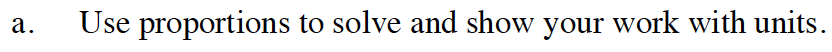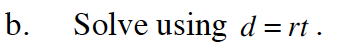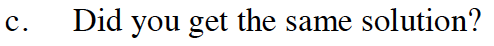Home > GB8I > Chapter cc46 > Lesson cc46.3.1 > Problem6-88

6-88.
1. A train traveled 90 miles in 1.5 hours. At the same rate how long will it take the train to travel 330 miles. Homework Help ✎

1. Use proportions to solve and show your work with units.

2. Solve using d = rt.

3. Did you get the same solution?$\frac{\text{?? miles}}{\text{?? hours}}=\frac{\text{?? miles}}{\text{?? hours}}$

$\frac{\text{90 miles}}{\text{1.5 hours}}=\frac{\text{330 miles}}{\textit{x}\text{ hours}} \ \ \text{Now finish solving this proportion.}$Since 90 miles is the distance (d) and 1.5 hours is the time (t), substitute in and solve for the rate (r).

$90=r*1.5$

$\frac{90}{1.5}=r$

$60=r$

$\text{Now use your rate of }\frac{60 \text{ miles}}{\text{hour}}\text{ to solve for time when the distance is 330 miles.}$Yes, both methods resulted in an answer of 5.5 hours to travel the 330 miles.